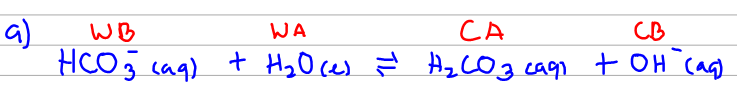# Problem: Write equations showing how each of the following weak bases ionizes water to form OH-.a) HCO3-b) CH3NH2Identify all of the phases in the answer.

###### FREE Expert Solution
85% (254 ratings)
###### FREE Expert Solution

The questions ask us to show the equation of how this base reacts with water. Water (H2O) will ionize into H+ and OH-. That H+ will go to the weak base to create its conjugate acid.85% (254 ratings)###### Problem Details

Write equations showing how each of the following weak bases ionizes water to form OH-.

a) HCO3-

b) CH3NH2

Identify all of the phases in the answer.

What scientific concept do you need to know in order to solve this problem?

Our tutors have indicated that to solve this problem you will need to apply the Identifying Acids and Bases concept. You can view video lessons to learn Identifying Acids and Bases. Or if you need more Identifying Acids and Bases practice, you can also practice Identifying Acids and Bases practice problems.

What is the difficulty of this problem?

Our tutors rated the difficulty ofWrite equations showing how each of the following weak bases...as medium difficulty.

How long does this problem take to solve?

Our expert Chemistry tutor, Dasha took 2 minutes and 20 seconds to solve this problem. You can follow their steps in the video explanation above.

What professor is this problem relevant for?

Based on our data, we think this problem is relevant for Professor Reed's class at University of Guelph.